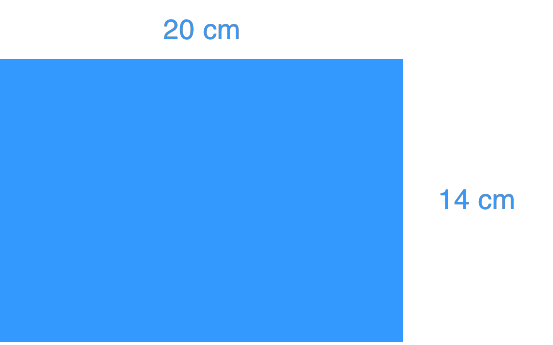# Squares and square roots#### All in One Place

Everything you need for better grades in university, high school and elementary.#### Learn with Ease

Made in Canada with help for all provincial curriculums, so you can study in confidence.#### Instant and Unlimited Help

0/2
##### Intros
###### Lessons
• What is a square root?
• How to find side lengths of squares using square roots?
• How to estimate non-perfect squares?
1. What is a perfect square?
0/10
##### Examples
###### Lessons
1. Find the prime factorization. Then, identify the perfect squares.
1. 53
2. 71
3. 196
2. Find the area of the square that has a side length of:
1. 15 cm
2. 21 cm
3. Calculate.
1. $\sqrt {16}$
2. $\sqrt {49}$
3. $\sqrt {2025}$

4.1. Find the area of the rectangle.
2. What is the side length of a square that has the same area as the rectangle above?
0%
##### Practice
###### Topic Notes
This lesson focuses on finding the square of a whole number and the square root of a perfect square. In order to do this, we will first learn how to do prime factorization, a method to find the prime factors of a number.